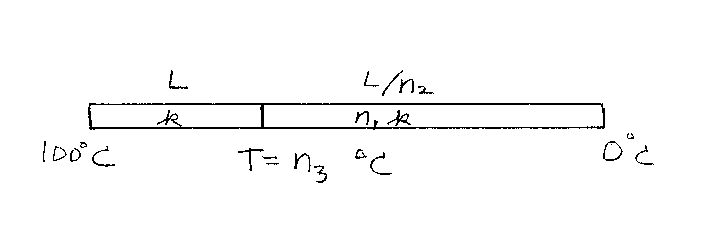Problem D16: Two long rods are attached at their ends as shown in the figure below. The left rod has a length of L and a thermal conductivity constant of k. The right rod has a length of L/n2 and a thermal conductivity constant equal to n1k. The left end is kept in boiling water at a temperature of 100 Deg C. The right end is kept in ice water at a temperature of 0 Deg C. What is the temperature at the spot where the two rods meet? If the temperature at the junction is T = n3 Deg C, what is n3? Note that n3 is in Deg C, but n1 and n2 are unitless.n1 = n2 = Input n3:

If you are currently in my class, you can record your grade by entering your name and student ID number (without the leading zeros) below and clicking on "record grade".
 First Name = Last Name = ID = Problem: